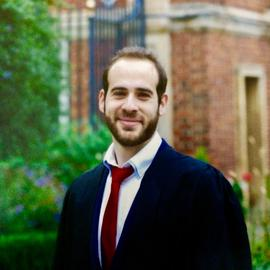# # 131 Puzzle from the New Scientist

Solution to #131 “The Paradise Club” puzzle from the New Scientist. This week (No 3352 - 18 September 2021) the New Scientist posted the following puzzle:

Down at The Paradise Club, Gus and Bart take it in turns to roll a pair of dice. The first person to score his favourite score for two dice wins, which means being treated to a drink by the other (the loser). They each favour a different prime number as a score with two dice and it so happens that their chances of getting their favourite score is the same for each.

What is that probability? If Gus goes first what are his chances of being bought a drink?

The maximum score (i.e. sum) when rolling two dice is 12. The prime numbers from 2 (the minimum score) to 12 are

$2, 3, 5, 7, 11$

Each score can be the result of the following dice combinations

2: {1, 1}

3: {2, 1}

5: {2, 3}, {4, 1}

7: {4, 3}, {5, 2}, {6, 1}

11: {6, 5}

For example, a score of 2 can only be achieved if both dice are {1, 1} (1 combination in total). A score of 3 can be achieved either with {2, 1} or {1, 2} (2 combinations in total). A score of 5 can be achieved with {2, 3} or {4, 1} or {3, 2} or {1, 4} (4 combinations in total).

The table below shows the total number of combinations for each score

ScoreTotal Combinations
21
32
54
76
112

The puzzle states that the chances of getting their favourite score is the same for Gus and Bart. Since each combination is equiprobable1 the only scores that result in the same chances are 3 and 11.

Now we need to find that probability. We can calculate it as

Probability = Number of favourable combinations/total number of combinations

We already know the number of favourable combinations (i.e. the number of combinations that will give the win) - it is 2. There are 2 ways to get a score of 3 or 11 (see table). The total number of combinations is 36. There are 36 possible outcomes when we roll two dice (6 from the first and 6 from the second die).

Hence, the probability of getting their favourite score is $$2/36 \text{ or } 1/18$$ and it is the same for both.

Then, we are asked: “If Gus goes first what are his chances of being bought a drink?” That is, what are his chances of winning? Gus wins if he rolls his favourite score or both Bart and Gus fail - in which case they continue the game. Let W denote the event that Gus wins. The probability of W is

P(W) = P(Gus rolls his favourite score ) + P(both fail)

This is usually called the addition rule2. We already know the first term, the probability of rolling his favourite score is $$1/18$$. The second term can be decomposed as

P(both fail) = P(Bart fails and Gus fails) =

P(Bart fails given that Gus fails) P(Gus fails)

P(Bart fails given that Gus fails) (1 - P(W))

To go from the first to the second line I used the multiplication rule3. Also, P(Gus fails) = 1 - P(Gus wins) = 1 - P(W). The first term in the last line (P(Bart fails given that Gus fails)) is equal to the probability that Bart fails, which is 1-P(Bart rolls his favourite score) = 1 - 1/18 = 17/18. So rewriting our previous equation we have

P(W) = P(Gus rolls his favourite score) + P(both fail)

P(W) = P(Gus rolls his favourite score) + P(Bart fails given that Gus fails) (1 - P(W))

P(W) = 1/18 + 17/18(1 - P(W))

P(W) = 18/35

The probability of Gus winning, if he goes first, is 18/35.

## Update 27/09/21

The solution from the New Scientist.

1. I have assumed that each die is fair, that is the occurrence of each number is equally likely.↩︎

2. The addition rule states that for two events A and B, the probability that either one or both events occur is P(A or B) = P(A) + P(B) - P(A and B). The last term, P(A and B), is the probability that both events occur. In our case, it is zero because we cannot have a score of 3 and 11 at the same time, they cannot occur together when rolling a pair of dice.↩︎

3. The multiplication rule states that the probability that both events occur is P(A and B) = P(A) P(B given A) or P(B) P(A given B). P(A given B) means the probability that event A occurs given event B has occurred.↩︎